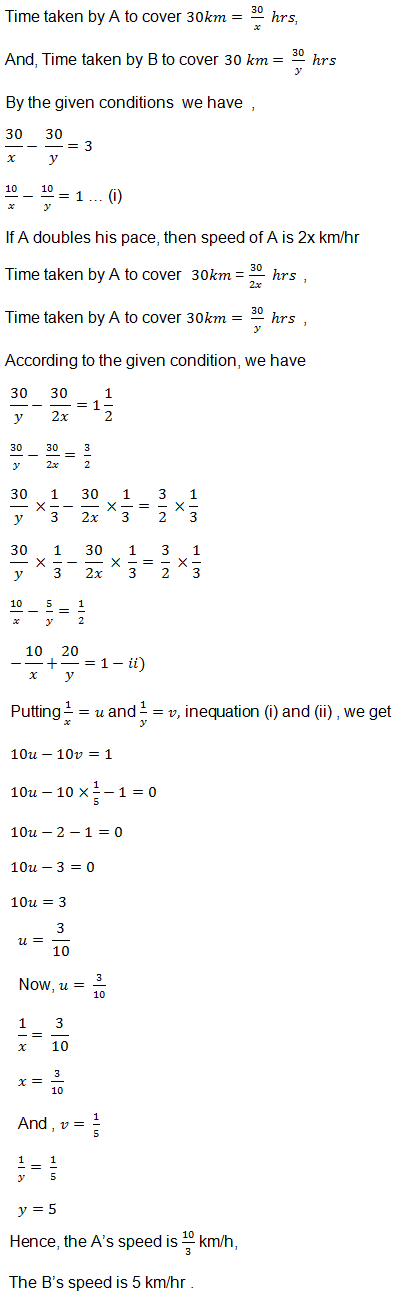## Chapter 3 Pair of Linear Equations in Two Variables R.D. Sharma Solutions for Class 10th Math Exercise 3.10

Exercise 3.10

1. Point A and B are 70 km. a part on a highway. A car starts from A and another car starts from B simultaneously. If they travel in the same direction, they meet in 7 hours, but if the travel towards each other, the meet in one hour. Find the speed of the two cars.

Solution

We have to find the speed of car
Let X and Y be two cars starting from points A and B respctively . Let the speed of car X be x km/hr ad that of car Y be y km/hr.
Case I: When two cars move in the same directions:
Suppose two cars meet at point Q , Then,
Distance travelled by car X = AQ
Distance travelled by car Y = BQ
It is given that two cars meet in 7 hours.
Therefore, Distance travelled by car X in 7 hours = 7x km
AQ = 7x
Distance traveled by car y in hours = 7 y km
BQ = 7Y
Clearly AQ – BQ = AB
7x – 7y = 70
Dividing both sides by common factor 7 we get,
x-y = 10….(i)
Case II : When two cars move in opposite direction
Suppose two cars meet at point. Then,
Distance travelled by car X = AP ,
Distance travelled by car Y = BP .
In this case, two cars meet in 1 hour
Therefore Distane travelled by car X in 1 Hour  = 1xKm
AP  = 1x
Distance travelled by car Y in 1 hour = 1y km
BP = 1y
From the above clearly,
AP + BP = AB
AP + BP = AB
By solving equation (i) and (ii), we get
x-y = 10
x+y = 70
2x = 80
x = 80 / 2
x = 40
Substituting x = 40 in equation (ii) we get
x + y = 70
40 + y = 70
y = 70 – 40
y = 30
Hence, the speed of car starting from point A is 40 km/hr.
The speed of car starting from point B is 30/hr .

2. A sailor goes 8 km downstream in 40 minutes and returns in 1 hours. Determine the speed of the sailor in still water and the speed of the current.

Solution

Let the speed of the sailor in still water x km/hr and the speed of the current be y km/hr
Speed upstream = (x-y) km/hr
Speed downstream = (x+y) km/hr
Now, Time taken to cover 8 km down stream = 8/x+y hhrs
Time taken to cover 8 km upstream = 8/x-y hrs
But, time taken to cover 8 km downstream in 40 minutes or 40/60 hours that is 2/3 hours
8/x+y = 2/3
8×3 = 2(x+y)
24 = 2x + 2y
Dividing both sides by common factor 2 we get
12 = x + y …(i)
Time taken to cover 8 km upstream in 1 hour
8/x-y = 1
8 = 1(x-y)
8 = x – y …(ii)
By solving  these equation (i) and (ii) we get
x+y = 12
x-y = 8
2x = 20
x = 20/2
x = 10
Substitute x = 10 in equation (i) we get
x+y = 12
10+y = 12
y = 12 – 10
y = 2
Hence the speed of sailor is 10km/hr
The speed of current is 2 km/hr .

3. The boat goes 30 km upstream and 44 km downstream in 10 hours. In 13 hours, it can go 40 km upstream and 55 km downstream. Determine the speed of stream and that of the boat in still water.

Solution

Let the speed of the boat in still water be x km/hr and the speed of the stream be y km/hr
Speed upstream = (x-y) km/hr
Speed down stream = (x+y) km/hr
Time taken to cover 30 km upstream = 30/x-y hrs
Time taken to cover 44 km downstream = 44/x+y hrs
But total time of journey is 10 hours
30/x-y + 44/x+y = 10 …(i)
Time taken to cover 40 km upstream = 40/x-y hrs
Time taken to cover 55 km downstream= 55/x+y hrs
In this case total time of journey is given to be 13 hours
Therefore, 40/x-y + 55/x+y = 13 …(ii)
Putting  1/x-y = u and 1/ x+y = v in equation (i) and (ii) we get
30u + 44v = 10
40u + 55v = 10
30u + 44v – 10 = 0 …(iii)
40u + 55v – 13 = 0 …(iv)
Solving these equations by cross multiplication we get4. A boat goes 24 km upstream and 28 km downstream in 6 hrs. It goes 30 km upstream and 21 km downstream in  6 fraction 1/2 hours . Find the speed of the boat in still water and also speed of the stream .

Solution

We have to find the speed of the boat in still water and speed of the stream
Let the speed of the boat in still water be x km/hr and the speed of the stream be y km/hr then
Speed upstream = (x-y) km/hr
Speed downstream = (x+y) km/hr5. A man walks a certain distance with certain speed. If he walks 1/2 km an hour faster, he takes 1 hour less. But, if he walks 1 km an hour slower, he takes 3 more hours. Find the distance covered by the man and his original rate of walking.

Solution

Let the actual speed of the train be x Km/hr and the actual time taken by y hours. Then,
Distance covered = speed × distance
= x × y
= xy …(i)
If the speed is increased by 1/2 km/hr , then time of journey is reduced by 1 hour i.e., when speed is (x + 1/2) km/hr , time of journey is (y-1) hours
Distance covered = xy km
-2x + y – 1 = 0 …(ii)
When the speed is reduced by 1 km/hr, then the  time of journey is increased by 3 hours i.e., when speed is (x-1) km/hr, time of journey is (y+3) hours
Distance covered = xy
xy = (x-1)(y+3)
xy = (x-1)(y+3)
xy = xy – 1y + 3x – 3
xy = xy + 3x – 1y – 3
3x – 1y – 3 = 0 …(iii)
Thus we obtain the following equations
-2x + 1y – 1 = 0
3x – 1y – 3 = 0
By using elimination method, we have
-2x + 1y – 1 = 0
3x – 1y – 3 = 0
1x – 4 = 0
x = 4
Putting the value x = 4 in equation (iii) we get
3x – 1y – 3 = 0
3 × 4 – 1y – 3 = 0
12 – 1y – 3 = 0
9 – 1y = 0
-1y = -9
-1y = -9
y = 9
Putting the value of x and y in equation (i) we get
Distance covered = xy
= 4 × 9
= 36 km
Hence, the distance is 36 km ,
The speed of walking is 4 km/hr .

6. A person rowing at the rate of 5 km/h in still water, takes thrice as much time in going 40 km downstream. Find the speed of the stream.

Solution

Speed of the boat in still water = 5 km/h
Let the speed of stream = x km/h
Speed of boat upstream = (5 – x) km/h
Speed of boat downstream = (5 + x) km/h
Time taken to row 40 km upstream = 40/5 – x
Time taken to row 40 km downstream = 40/5 + x
According to the given condition,
40/5 – x = 3(40/5+x)
⇒ 1/5 – x = 3/5 + x
⇒ 5 + x = 15 – 3x
⇒ 4x = 10
⇒ x = 10/4 = 2.5 km/h
Therefore, the speed of the stream is 2.5 km/h .

7. Ramesh travels 760 km to his home partly by train and partly by car. He takes 8 hours if he travels 160 km. by train and the rest by car. He takes 12 minutes more if the travels 240 km by train and the rest by car. Find the speed of the train and car respectively.

Solution

Let the speed of the train be x km/hour that of the car be y km/hr, we have the following cases
Case I: When Ramesh travels 760 Km by train and the rest by car8. A man travels 600 km partly by train and partly by car. If the covers 400 km by train and the rest by car, it takes him 6 hours 30 minutes. But, if the travels 200 km by train and the rest by car, he takes half an hour longer. Find the speed of the train and that of the car.

Solution

Let the speed of the train be x km/hr that of the car be y km/hr, we have the following cases:
Case I: When a man travels 600Km by train and the rest by car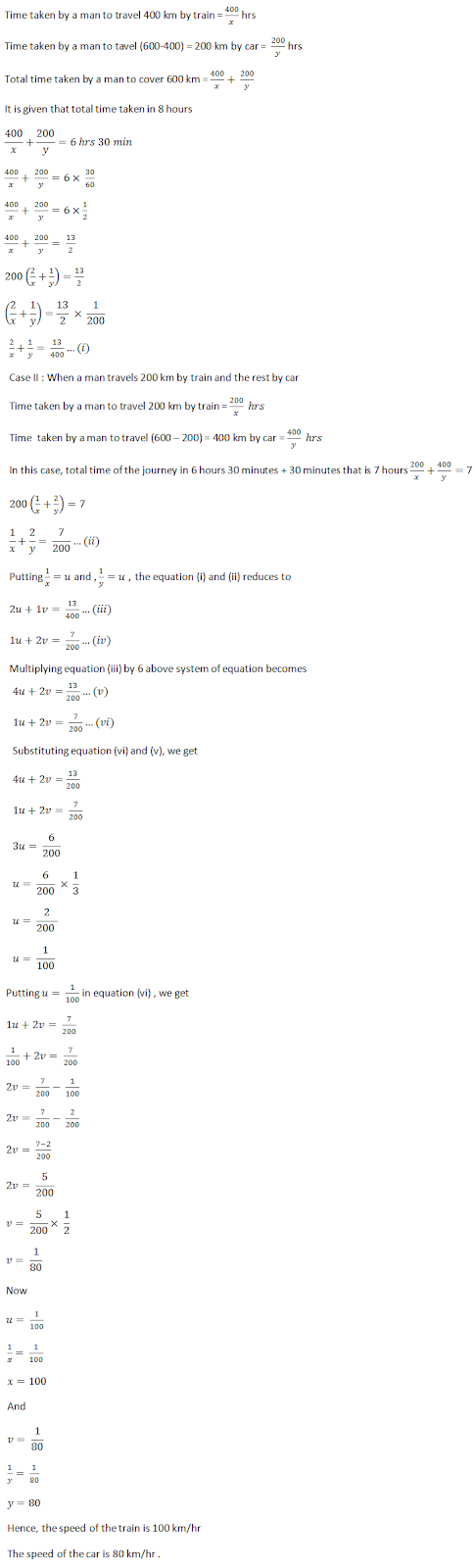9. Places A and B are 80 km apart from each other on a highway. A car starts from A and other from B at the same time. If they move in the same direction, they meet in 8 hours and if they move in opposite directions, they meet in 1 hour and 20 minutes. Find the speeds of the cars.

Solution

Let x and y be two cars starting from points A and B respectively.
Let the speed of the car X be x km/hr and that of the car Y be y km/hr.

Case I: When two cars move in the same directions:
Suppose two cars meet at point Q, then,
Distance travelled by car X = AQ
Distance travelled by car Y = BQ
It is given that two cars meet in 8 hours.
Distance travelled by car X in 8 hours = 8x km
AQ = 8x
Distance travelled by car Y in 8 hours = 8y km
BQ = 8y
Clearly AQ – BQ = AB
8x – 8y = 80
Both sides divided by 8, we get
x – y = 10 …(i)

Case II: When two cars move in opposite direction
Suppose two cars meet at point P, then ,
Distance travelled by X car X = AP
Distance travelled by Y car Y = BP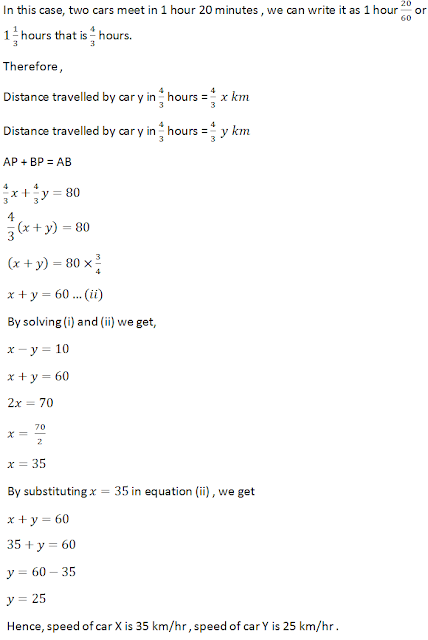10. A boat goes 12 km upstream and 40 km downstream in 8 hours. I can go 16 km upstream and 32 km downstream in the same time. Find the speed of the boat in still water and the speed of the stream.

Solution

We have to find the speed of the boat in still water and speed of the stream
Let the speed of the boat in still water be x km/hr and the speed of the stream be y km/hr
Speed upstream = (x-y) km/hr
Speed downstream = (x+y) km/hr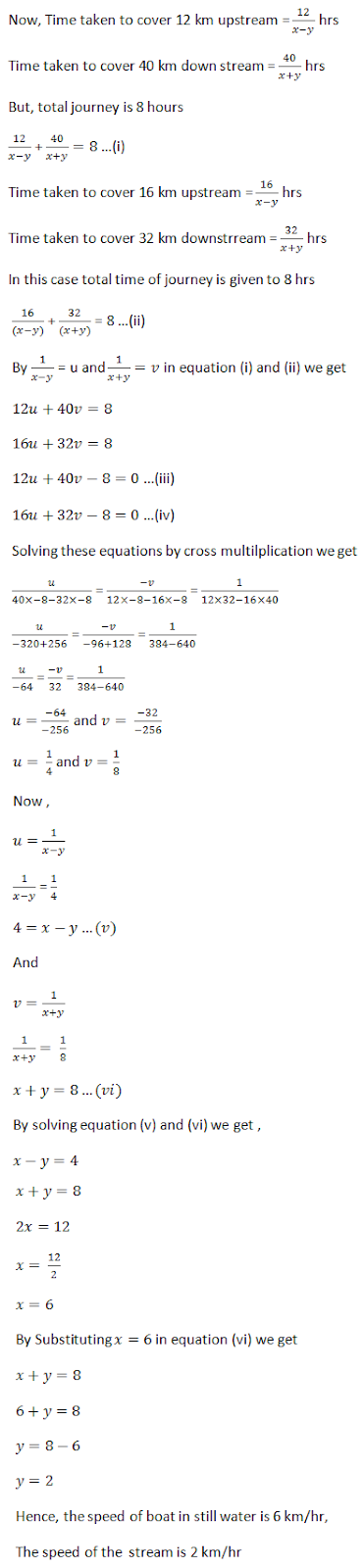11. Roohi travels 300 km to her home partly by train and partly by bus. She takes 4 hours if she travels 60 km by train and the remaining by bus. If she travels 100 km by train and the remaining by bus, she takes 10 minutes longer. Find the speed of the train and the bus separately.

Solution

Let the speed of the train be x km/hour that of the bus be y km/hr, we have the following cases

Case I: When Roohi travels 300 Km by train and the rest by bus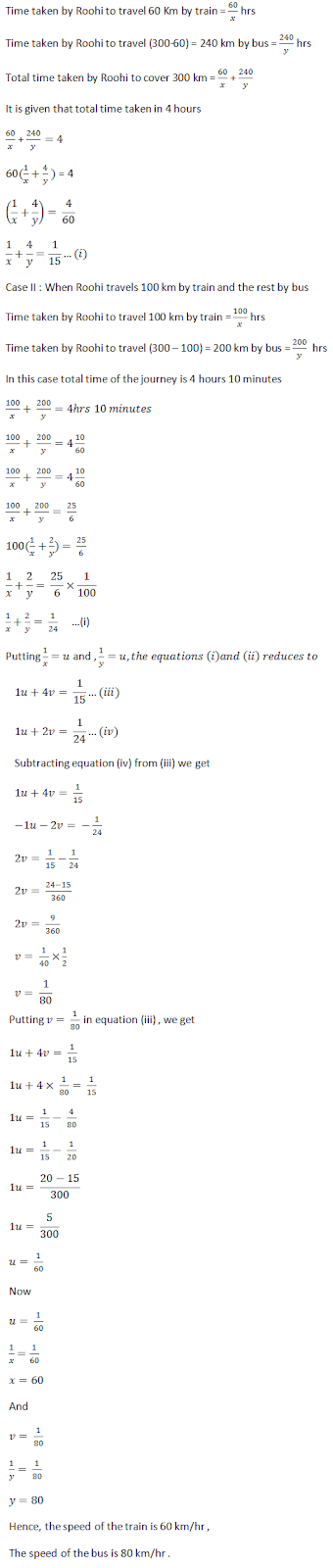12. Ritu can row downstream 20 km in 2 hours, and upstream 4 km in 2 hours. Find her speed of rowing in still water and the speed of the current.

Solution

Let the speed of rowing in still water be x km/hr and the speed of the current be y km/hr
Speed upstream = (x-y) km/hr
Speed downstream = (x+y) km/hr
Now,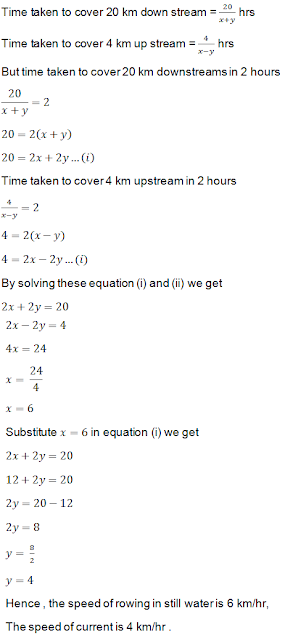13. A motor boat can travel 30 km upstream and 28 km downstream in 7 hours. It can travel 21 km upstream and return in 5 hours. Find the speed of the boat in still water and the speed of the stream.

Solution

Let the speed of the boat in still water be x km/h and the speed of the stream be y km/h.
Speed of boat upstream = x − y
Speed of boat downstream = x + y
It is given that, the boat travels 30 km upstream and 28 km downstream in 7 hours.
∴ 30/x-y + 28/x+y = 7
Also, the boat travels 21 km upstream and return in 5 hours .
∴ 21/x-y + 21/x+y = 5
Let 1/x-y = u and 1/x+y = v.
So, the equation becomes
30u + 28v = 7 …(i)
21u + 21v = 5 …(ii)
Multiplying (i) by 21 and (ii) by 30, we get
630u + 588v = 147 …(iii)
630u + 630v = 150 …(iv)
Solving (iii) and (iv), we get
v = 1/14 and u = 1/6
But, 1/x-y = u and 1/x+y = v
So,
1/x-y = 1/6 and 1/x+y = 1/14
⇒ x – y = 6 and x + y = 14
Solving these two equations , we get
x = 10 and y = 4
So, the speed of boat in still water = 10 km/h and speed of stream = 4 km/h.

14. Abdul travelled 300 km by train and 200 km by taxi, it took him 5 hours 30 minutes. But if he travels 260 km by train and 240 km by taxi he takes 6 minutes longer. Find the speed of the train and that of the taxi.

Solution

Let the speed of the train be x km/hour that of the taxi be y km/hr, we have the following cases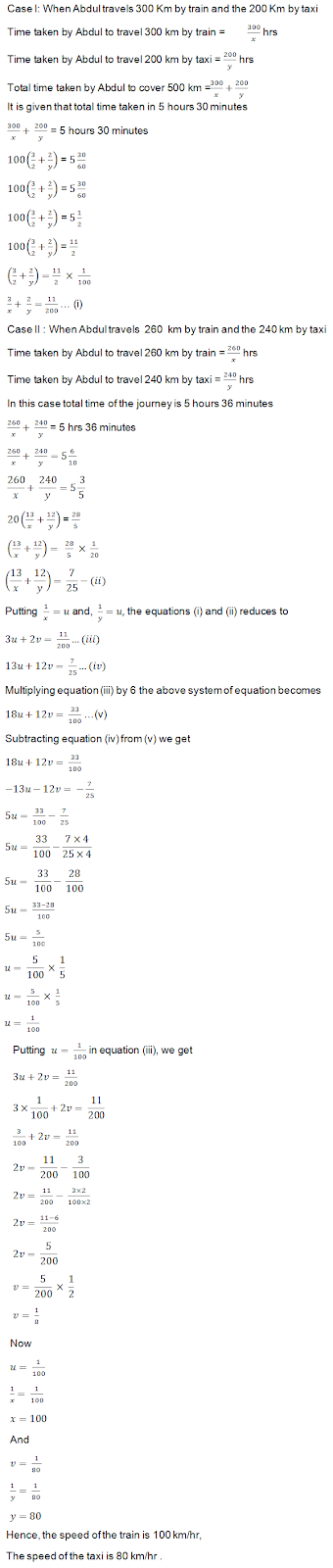15. A train covered a certain distance at a uniform speed. If the train could have been 10km/hr. faster, it would have taken 2 hours less than the scheduled time. And, if the train were slower by 10km/hr; it would have taken 3 hours more than the scheduled time. Find the distance coverec by train.

Solution

Let the actual speed of the train 1 km/hr and the actual time taken by y hours . Then,
Distance =  speed × Time
Distance covered = (xy)km …(i)
If the speed is increased by 10 km/hr , then time of journey is reduced by 2 hours
When speed is (x+10) km/hr, time of journey is (y-2)hours
Distance covered = (x+10)(y-2)
xy = (x+10)(y-2)
xy = xy + 10y – 2x – 20
-2x + 10y – 20 = 0
-2x + 3y – 12 = 0 – (ii)
When the speed is reduced  by 10 km/hr , then the time of journey is increased by 3 hours when speed is (x-10) km/hr, time of journey is (y+3) hours
Distance overed = (x – 10)(y+3)
xy = (x-10)(y+3)
xy = xy – 10y + 3x – 30
0 = -10y + 3x – 30
3x – 10y – 30 = 0 …(iii)
Thus, we obtain the following system of equations   :
-x + 5y – 10 = 0
3x – 10y – 30 = 0
By using cross multilication, we haveHence, the length pf the journey is 600 km .

16. Places A and B are 100 km apart on a highway. One car starts from A and another from B at the same time. If the cars travel in the same direction at different speeds, they meet in 5 hours. If they travel towards each other, they meet in 1 hour. What are the speeds of two cars?

Solution

Let x and y be two cars starting from points A and B respectively.
Let the speed of the car X be x km/hr and that of the car Y be y km/hr.

Case I: When two cars move in the same directions:
Suppose two cars meet at point Q, then,
Distance travelled by car X=AQ
Distance travelled by car Y=BQ
It is given that two cars meet in 5 hours.
Distance travelled by car X in 5 hours  =  5x km AQ = 5x
Distance travelled by car y  in 5 hours  = 5y km BQ = 5y
Clearly AQ – BQ = AB 5x – 5y = 100
Both sides divided by 5, we get x – y = 20 …(i)

Case II : When two cars move in opposite direction
Suppose two cars meet at point P, then ,
Distance travelled by X car X = AP
Distance travelled by Y car Y = BP
In this case, two cars meet in 1 hour
Therefore ,
Distance travelled by car x in 1 hours = 1x km
Distance travelled by car y in 1 hours = 1y km
AP + BP = AB
1x + 1y = 100
x + y = 100
x + y = 100 – (ii)
By solving (i) and (ii) we get ,
x-y = 20
x+y = 100
2x = 120
x = 120 / 2
x = 60
By substituting x = 60  in equation (ii), we get
x + y = 100
60 + y = 100
y = 100 – 60
y = 40
Hence, speed of car X is 60 km/hr , speed of car Y is 40 km/hr .

17. While covering a distance of 30 km. Ajeet takes 2 hours more than Amit. If Ajeet doubles his speed, he would take 1 hour less than Amit. Find their speeds of walking.

Solution

Let the speed of Ajeet and Amit be x Km/hr respectively. Then,
Time taken by Ajeet to cover 30 km = 3 /x hrs
Time taken by Amit to cover 30 km = 30/y hrs
By this given condition , we have
30/x – 30/y = 2 …(i)
If Ajeet doubles his speed , then speed of Ajeet is 2x km/hr
Time taken by Ajeet to cover 30 km = 30/2x hrs
Time taken by Amit to cover 30 km = 30/y hrs
According to the given condition, we have
-15x + 30/y = 1 …(ii)
Putting 1/x = u and 1/y = v , in equation (i) and (ii), we get
30u – 30v = 2 …(iii)
-15u + 30v = 1 …(iv)
Adding equations (iii) and (iv), we get
30u – 30v = 2
-15u + 30v = 1
15u = 3
u = 15/3
u = 1/5
Putting u = 1/5 in equation (iii) , we get
30u – 30v = 2
30 × 1/5 – 30v = 2
6 – 30v = 2
-30v = 2 – 6
-30v = -4
v = - 4/-30
v = 2/15
Now, u = 1/5
1/x = 1/5
x = 5
and v = 2/15
1/y = 2/15
y = 15/2
y = 7.5
Hence the speed of Ajeet is 5 km/hr
The speed of Amit is 7.5 km/hr

18. A takes 3 hours more than B to walk a distance of 30 km. But, if A doubles his pace(speed) he is ahead of B by 1½ hours. Find the speeds of A and B.

Solution

Let the speed of A and B be x Km/hr and y Km/hr respectively. Then,